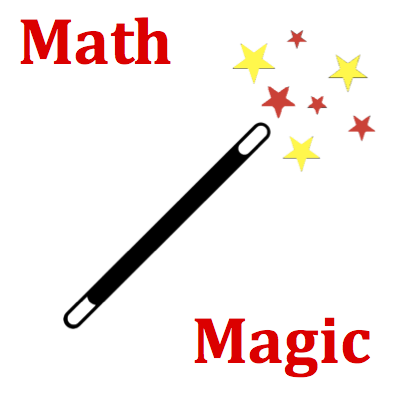# Circular and Satellite Motion - Mission CG7 Detailed HelpAn object of mass 'm' is located a distance 'R' from the center of a planet with mass 'M.' The acceleration of gravity of the mass 'm' at this location is 12 m/s/s. Suppose that the mass of the object is doubled to '2m' and the separation distance is halved to '0.5R.' The new acceleration of gravity value for the mass and the new location would be ____ m/s/s. Note: The numerical values in your question were randomly selected and may differ from those shown above.Acceleration of Gravity or Gravitational Field Strength: The acceleration of gravity at any given location near or above a planet's surface is often referred to as the gravitational field constant of that planet. Such acceleration values are directly proportional to the planet's mass and inversely proportional to the square of the distance from the planet's center.This question explores the affect of changing object mass and changing separation distance upon the acceleration of gravity. The acceleration of gravity is dependent upon an object's location and would be affected by changes in its distance from a planet's center. But don't be fooled! The acceleration of gravity of an object is not affected by the mass of the object.This question targets the relationship between separation distance and the acceleration of gravity. Gravitational acceleration is inversely proportional to the square of the separation distance. The inverse nature of the law means that if the distance is increased, then the gravitational acceleration will be decreased. The inverse square nature of the law means the acceleration value will be decreased by the square of the factor by which the separation distance is increased. So if the separation distance becomes two times bigger, then the gravitational acceleration will become four times (22) smaller. The new gravitational acceleration would be one-fourth the original value. If the separation distance becomes three times bigger, then the gravitational acceleration will become nine times (32) smaller. The new gravitational acceleration would be one-ninth the original value. If the separation distance becomes one-half the size, then the gravitational acceleration will be four times (22) large. The new gravitational acceleration would be four times the original value.